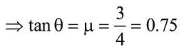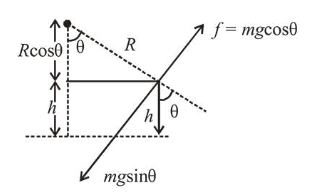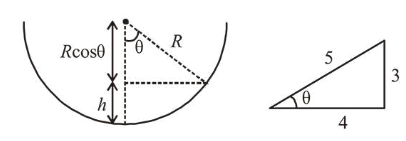# An insect is at the bottom of a hemispherical ditch of radiusQuestion:

An insect is at the bottom of a hemispherical ditch of radius $1 \mathrm{~m}$. It crawls up the ditch but starts slipping after it is at height $h$ from the bottom. If the coefficient of friction between the ground and the insect is $0.75$, then $h$ is : $\left(\mathrm{g}=10 \mathrm{~ms}^{-2}\right)$

1. (1) $0.20 \mathrm{~m}$

2. (2) $0.45 \mathrm{~m}$

3. (3) $0.60 \mathrm{~m}$

4. (4) $0.80 \mathrm{~m}$

Correct Option: 1

Solution:

(1) For balancing, $m g \sin \theta=f=\mu m g \cos \theta$$h=R-R \cos \theta=R-R\left(\frac{4}{5}\right)=\frac{R}{5}$

$\therefore h=\frac{R}{5}=0.2 \mathrm{~m}$        $[\because$ radius, $R=1 \mathrm{~m}]$